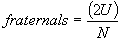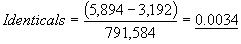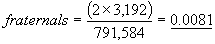## Appendix D - TWIN AND MULTIPLE BIRTH FREQUENCIES

Twins

The proportions of identical and fraternal twins in a population can be estimated by the Weinberg method from the relative numbers of like-sexed and unlike-sexed twins (Bulmer, 1970). Thus, if L and U are the numbers of like-sexed and unlike-sexed twin maternities, the identical and fraternal twinning rates are estimated as:

Equation 6and

Equation 7For example, in England and Wales in 1960, out of 791,584 pregnancies there were 9,086 twin pregnancies, of which 5,894 were like-gendered and 3,192 were unlike-gendered. The two types of twinning rates, therefore, are estimated as follows:i.e. 3.4 per 1,000 pregnancies, andi.e. 8.1 per 1,000 pregnancies.

Multiple Births

In a previous section it was noted that multiple births of more than two children were so rare as not to merit separate codes. The following figures were presented by Bulmer (1970):

Number of Multiple Births per Million Pregnancies

 Twins 12,500 (England and Wales, 1946 - 1955) Triplets 109 (England and Wales, 1938 - 1962) Quadruplets 1.7 (Combined data from England and Wales, U.S. A., Italy and France)

Since 1970 the number of multiple births has increased due to fertility treatments, but is still relatively low.

The Encyclopaedia Britannica (2003 ed.) gives the following method for calculating the approximate incidence of naturally-occurring multiple births:If the frequency of twins is 1/80 then the proportion of triplets will be (1/80)2 = 1/6400, quadruplets (1/80)3 = 1/512,000, quintuplets (1/80)4 = 1/40,960,000 and so on.